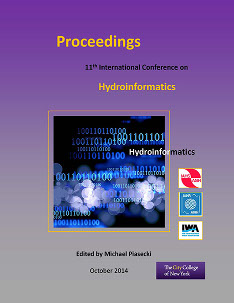## International Conference on Hydroinformatics

Presentation

8-1-2014

#### Abstract

We present a novel nDP (nested dynamic programming) algorithm for multipurpose reservoir optimization. The nDP algorithm is built from two algorithms: 1) dynamic programming (DP) and 2) nested optimal water allocation algorithm implemented with Simplex and quadratic Knapsack. The main objective function is to minimize the sum of weighted square deviations of each objective over the whole time horizon. Each objective is described with its water demand or target and its weights at each time step. The nDP algorithm begins with the DP optimization algorithm that at each DP transition executes the nested optimal water allocation algorithm. The optimal water allocation algorithm distributes the entire reservoir release between the users and calculates the users' weighted square deviations. With the user releases and the reservoir storage variables it is possible to create more complex objective functions e.g. hydropower and afterward calculate the main objective function. The nDP can solve a multi-objectives optimization problem without significant increase in the algorithm complexity and the computational expenses and provides a framework for including other nested optimization algorithms. The nDP is coded in Java as a prototype application and was successfully tested on the Knezevo reservoir with eight objectives and six decision variables. The nDP is computationally very efficient and it can handle dense and irregular variable discretization. The nDP was compared with a classical DP and a two-stage DP algorithm. The two-stage DP algorithm in the first stage calculates the optimal release and in the second stage distributes the release between users and determines the objective function. The comparison demonstrated that the nDP is a better optimization algorithm than the two-stage DP algorithm while the classical DP is not a feasible solution.